# AP Chemistry : VSEPR and Bond Hybridization

## Example Questions

← Previous 1 3 4 5

### Example Question #1 : Vsepr And Bond Hybridization

What is the molecular shape of the following molecule?

SF6

Possible Answers:

octahedral

trigonal bipyramidal

seesaw

linear

hexahedral

Correct answer:

octahedral

Explanation:

when a central atom of a molecule has 6 electron domains coming off of it (none of which are lone pairs of electrons), it is considered octahedral

### Example Question #1 : Vsepr And Bond Hybridization

What is the shape of the SO2 molecule?

Possible Answers:

Linear

Bent

Square planar

Octahedral

Trigonal planar

Correct answer:

Bent

Explanation:

The answer to this question can be determined by drawing a Lewis structure of the molecule. The S atom is central, with an O atom on each side. Adding in double bonds to each O atom and placing lone pairs on the O atoms give them each an octet, which leaves 2 more lone pairs of electrons to go on the S atom, creating a bent shape.

### Example Question #3 : Vsepr And Bond Hybridization

VSEPR theory predicts that a BF3 molecule will be which of the following shapes?

Possible Answers:

Tetrahedral

Square planar

Bent

Trigonal planar

Correct answer:

Trigonal planar

Explanation:

Molecules with three atoms around a central atom such as BF3 are trigonal planar because electron repulsion is minimized by positioning the three attachments toward the corners of an equilateral triangle.

### Example Question #4 : Vsepr And Bond Hybridization

Which of the following is not a characteristic of a preferred Lewis structure?

Possible Answers:

Less separation between opposite charges

Negative formal charges placed on more electronegative atoms

Multiple bonds between atoms

Small or no formal charges

Correct answer:

Multiple bonds between atoms

Explanation:

Multiple bonds, although they may be stronger, are not necessarily favored in a Lewis structure. It is dependent on the atoms involved. Thus, this is not a criterion used in determining a preferred Lewis structure.

### Example Question #5 : Vsepr And Bond Hybridization

Consider the following reaction, which occurs at high temperatures with a silver catalyst: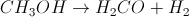What is the change in the geometry of the carbon atom between the reactant and the product?

Possible Answers:

Square planar to trigonal pyramidal

Square planar to trigonal planar

Tetrahedral to trigonal pyramidal

Tetrahedral to trigonal planar

Trigonal planar to tetrahedral

Correct answer:

Tetrahedral to trigonal planar

Explanation:

In the reactant,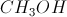, the carbon is bound to three hydrogens and one oxygen, and it has no lone pairs, so it has a tetrahedral geometry. In the product,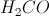, the carbon is bound to two hydrogens and one oxygen, so it must be double bonded to the oxygen in order to have a complete octet. Therefore, it has a trigonal planar geometry.

### Example Question #6 : Vsepr And Bond Hybridization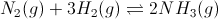Figure 1: Ammonia gas formation and equilibrium

What type of geometry does ammonia exhibit?

Possible Answers:

Octahedral

Trigonal pyramidal

Tetrahedral

Bent

See-saw

Correct answer:

Trigonal pyramidal

Explanation:

Ammonia exhibits a tetrahedral electron pair geometry. It has three bonded pairs (between nitrogen and each hydrogen), and one lone pair (on nitrogen). This combination forms a trigonal pyramidal molecular geometry.

### Example Question #1 : Vsepr And Bond Hybridization

For which of the following molecules are the molecular and electronic geometries NOT the same?

Possible Answers:

SO3

PCl3

BeBr2

SiH4

Correct answer:

PCl3

Explanation:

The molecular geometry refers to the shape of the molecule's atoms, while the electronic geometry refers to the shape of the molecule's atoms and lone pair electrons. PCl3 has a trigonal pyramidal molecular geometry and a tetrahedral electronic geometry. All other answers have no lone pairs, and therefore have the same molecular and electronic geometries.

### Example Question #8 : Vsepr And Bond Hybridization

What is the molecular geometry of ammonia (NH3)?

Possible Answers:

Tetrahedral

Trigonal pyramidal

See-saw

Trigonal planar

Correct answer:

Trigonal pyramidal

Explanation:

This answer relies on remembering that ammonia has a lone electron pair in order to fill its valence shell. Without this lone pair, trigonal planar would be correct, however, since this electron pair is present, it repels the three hydrogens further away from a planar configuration. This results in a trigonal pyramidal shape.

### Example Question #2 : Vsepr And Bond Hybridization

Which of the following compounds has an octahedral geometry?

Possible Answers: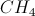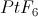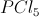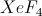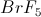Correct answer:Explanation:

The only answer choice that works is platinum hexafluoride. The platinum molecule is able to bind six fluorine molecules due to the availability of d orbitals in its valence shell. The octahedral shape looks like two pyramids with four sides each that have been stuck together by their bases. We can imagine the platinum at the middle with the six fluorines at each of the vertices of the pyramids. To have the octahedral shape, a molecule must have a central atom and six constituents.

### Example Question #1 : Vsepr And Bond Hybridization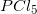has a trigonal bipyramidal geometry. What are the two bond angles present in?

Possible Answers: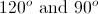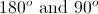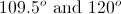Correct answer:Explanation:

In order for the five chlorine atoms around phosphorus to be distanced from one another, they will orient themselves in a trigonal bipyramidal fashion. This results in trigonal planar geometry in the x-axis. There is also one chlorine atom sticking up out of the plane, and one chlorine atom sticking down out of the plane. This results in the chlorine atoms in the trigonal planar geometry being 120o away from each other, and the chlorine atoms in the y-axis being 90o away from the chlorine atoms in the trigional planar geometry.

← Previous 1 3 4 5

### All AP Chemistry Resources